Courses

# Test: Waves- 2

## 25 Questions MCQ Test Physics Class 11 | Test: Waves- 2

Description
This mock test of Test: Waves- 2 for Class 11 helps you for every Class 11 entrance exam. This contains 25 Multiple Choice Questions for Class 11 Test: Waves- 2 (mcq) to study with solutions a complete question bank. The solved questions answers in this Test: Waves- 2 quiz give you a good mix of easy questions and tough questions. Class 11 students definitely take this Test: Waves- 2 exercise for a better result in the exam. You can find other Test: Waves- 2 extra questions, long questions & short questions for Class 11 on EduRev as well by searching above.
*Multiple options can be correct
QUESTION: 1

### To observe a stationary interference pattern formed by two light waves, it is not necessary that they must have :

Solution:
*Multiple options can be correct
QUESTION: 2

### In a YDSE apparatus, we use white light then :

Solution:
*Multiple options can be correct
QUESTION: 3

### If the source of light used in a Young's Double Slit Experiment is changed from red to blue, then

Solution:
*Multiple options can be correct
QUESTION: 4

In a Young's double slit experiment, green light is incident on the two slits. The interference pattern is observed on a screen. Which of the following changes would cause the observed fringes to be more closely spaced ?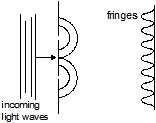Solution:
*Multiple options can be correct
QUESTION: 5

In a Young's double-slit experiment, let A and B be the two slits. A thin film of thickness t and refractive index m is placed in front of A. Let b = fringe width. The central maximum will shift :

Solution:
*Multiple options can be correct
QUESTION: 6

In the previous question, films of thicknesses tA and tB and refractive indices mA and mB, are placed in front of A and B respectively. If mAtA = mBtB, the central maximum will :

Solution:
*Multiple options can be correct
QUESTION: 7

In a YDSE, if the slits are of unequal width.

Solution:
*Multiple options can be correct
QUESTION: 8

If one of the slits of a standard YDSE apparatus is covered by a thin parallel sided glass slab so that it transmit only one half of the light intensity of the other, then

Solution:
*Multiple options can be correct
QUESTION: 9

To make the central fringe at the centre O, a mica sheet of refractive index 1.5 is introduced. Choose the correct statements (s).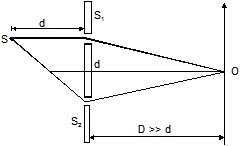Solution:
*Multiple options can be correct
QUESTION: 10

Choose the correct statement(s) related to the wavelength of light used

Solution:
*Multiple options can be correct
QUESTION: 11

If the distance D is varied, then choose the correct statement(s)

Solution:
*Multiple options can be correct
QUESTION: 12

If the distance d is varied, then identify the correct statement

Solution:
*Multiple options can be correct
QUESTION: 13

In an interference arrangement similar to Young's double-slit experiment, the slits S1 & S2 are illuminated with coherent microwave sources, each of frequency 106 Hz. The sources are synchronized to have zero phase difference. The slits are separated by a distance d = 150.0 m. The intensity I(q) is measured as a function of q, where q is defined as shown. If I0 is the maximum intensity then I(q) for 0 £ q £ 90° is given by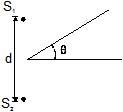Solution:

I=I0cos2(πdtanθ/ λ)/
= I0cos2(πx150xtanθ/3x(108/106)
=I0cos2(πtanθ/2)
θ=30o
I= I0cos2 ((π/2√3)
I=I0/2
θ=0
I=I0cos2(0) [cos0=1]
I= I0

*Multiple options can be correct
QUESTION: 14

In a standard YDSE appratus a thin film (m = 1.5, t = 2.1 mm) is placed in front of upper slit. How far above or below the centre point of the screen are two nearest maxima located ? Take D = 1 m, d = 1mm, l = 4500 Å. (Symbols have usual meaning)

Solution: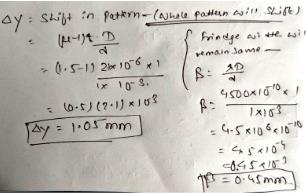*Multiple options can be correct
QUESTION: 15

Consider a case of thin film interference as shown. Thickness of film is equal to wavelength of light is m2.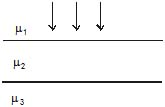Solution:
*Multiple options can be correct
QUESTION: 16

Figure shown plane waves refracted for air to water using Huygen's principle a, b, c, d, e are lengths on the diagram. The refractive index of water wrt air is the ratio.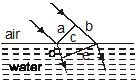Solution: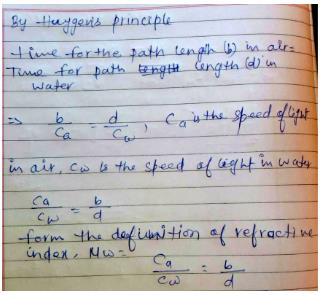QUESTION: 17

Two point source separated by

d = 5mm emit light of wavelength l = 2mm in phase. A circular wire of radius 20mm is placed around

the source as shown in figure.

Solution:
QUESTION: 18

Two monochromatic (wavelength = a/5) and coherent sources of electromagnetic waves are placed on the x-axis at the points (2a, 0) and (_a, 0). A detector moves in a circle of radius R(>>2a) whose centre is at the origin. The number of maximas detected during one circular revolution by the detector are

Solution:
QUESTION: 19

Two coherent narrow slits emitting light of wavelength l in the same phase are placed parallel to each other at a small separation of 3l. The light is collected on a screen S which is placed at a distance D (>> l) from the slits. The smallest distance x such that the P is a maxima.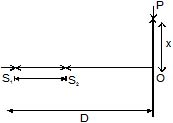Solution:
QUESTION: 20

A beam of light consisting of two wavelengths 6500 Å and 5200 Å is used to obtain interference fringes in Young's double slit experiment. The distance between slits is 2mm and the distance of screen from slits is 120 cm. What is the least distance from central maximum where the bright due to both wavelength coincide ?

Solution:

If n is least number of fringes of λ1(=6500A∘) which are coincident with (n+1) of smaller wavelength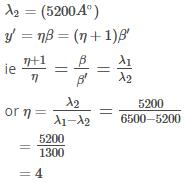also β=λD/d
substituting values for wavelength,D and d we get β = 0.039cm
So,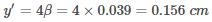Hence b is the correct answer.

QUESTION: 21

In Young's double slit experiment, the two slits act as coherent sources of equal amplitude A and wavelength l. In another experiment with the same setup the two slits are sources of equal amplitude A and wavelength l but are incoherent. The ratio of the average intensity of light at the midpoint of the screen in the first case to that in the second case is

Solution:
QUESTION: 22

A monochromatic light source of wavelength l is placed at S. Three slits S1, S2 and S3 are equidistant from the source S and the point P on the screen. S1P _ S2P = l/6 and S1P _ S3P = 2l/3. If I be the intensity at P when only one slit is open, the intensity at P when all the three slits are open is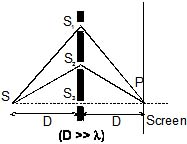Solution:
QUESTION: 23

In the figure shown in YDSE, a parallel beam of light is incident on the slit from a medium of refractive index n1. The wavelength of light in this medium is l1. A transparent slab to thickness `t' and refractive index n3 is put infront of one slit. The medium between the screen and the plane of the slits is n2. The phase difference between the light waves reaching point `O' (symmetrical, relative to the slits) is :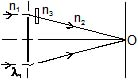Solution:
QUESTION: 24

In a YDSE experiment if a slab whose refractive index can be varied is placed in front of one of the slits then the variation of resultant intensity at mid-point of screen with `m' will be best represented by (m ³ 1). [Assume slits of equal width and there is no absorption by slab]

Solution:
QUESTION: 25

In a YDSE with two identical slits, when the upper slits is covered with a thin, perfectly transparent sheet of mica, the intensity at the centre of screen reduces to 75% of the initial value. Second minima is observed to be above this point and third maxima below it. Which of the following can not be a possible value of phase difference caused by the mica sheet.

Solution: